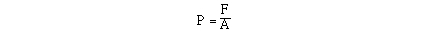### Pressure is a measure of the force of an object, or collection of particles, on a given area. Another way of saying the same thing, is pressure is the force exerted on an object divided by the area over which the force is distributed. Mathematically, we would write this relationship in the following way;